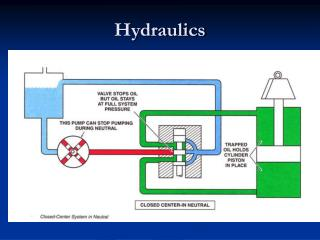DownloadDownload PresentationHydraulics

# Hydraulics

Télécharger la présentation## Hydraulics

- - - - - - - - - - - - - - - - - - - - - - - - - - - E N D - - - - - - - - - - - - - - - - - - - - - - - - - - -
##### Presentation Transcript

1. Hydraulics

2. Hydraulics • Pumps convert mechanical to hydraulic power. • Formulas: Flow: Qap = (DpNp/231)eVp where Qap = actual flow from pump (gph) Dp = pump displacement (in.3/rev) Np = pump speed (rpm) eVp = volumetric efficiency of pump 1 gal = 231 in3

3. Hydraulics Torque: Tsp = Δp Dp/(2π× 12 × eTp) where Tsp = shaft torque of pump (lb∙ft) Δp = pressure across pump (psi) eTp = torque efficiency of pump Hydraulic power: Phyp = Δp Qap/1714 (hp) Shaft power: Psp = Phyp /ePp = 2π Tsp Np/33000 where ePp = power(overall) efficiency = eVp × eTp

4. Hydraulic pump example: • Problem # 114 in practice problems. Pump flow desired is 20 gpm with a motor speed between 1700 and 2200 rpm. Choose the smallest pump. Qap = (DpNp/231)eVp Solve for Dp using trial and error. For pump JT02: Dp = 231 in.3/gal×20 gpm/(2200 rpm×0.91) = 2.31 in.3/rev > 2.0 in.3/rev and won’t pump enough at fastest speed. For pump JT03: Dp = 231×20/(1700×0.91) = 2.99 in.3 and okay since lowest speed won’t over pump and we can set speed to deliver 20 gpm using the 3.0 in.3/rev.

5. Hydraulic pump practice problem: Calculate the shaft power to drive the pump for JT03 with data from table of Qap = 20 gpm, Δp = 3000 psi system pressure – 100 psi pump input prressure, and ePp = 84%.

6. Hydraulic pump practice problem: • Calculate the shaft power to drive the pump for JT03. Pump flow is 20 gpm, system pressure is 3000 psi, input pressure at pump is 100 psi, and pump overall efficiency is 0.84. Psp = Phyp /ePp = [20 gpm× (3000-100)psi]/(1714×0.84) = 40.3 hp

7. Hydraulic Cylinder Hydraulic cylinder converts hydraulic power to mechanical power.

8. Hydraulics • Cylinders • Formulas Force to extend: Fext = ppisApis – prod(Apis-Arod) (lb) where ppis = piston pressure (psi) Apis = piston area (in.2) prod = rod pressure (psi) ≈ 0 Arod = rod area (in.2) Force to retract: Fret = prod(Apis-Arod) – ppisApis where ppis ≈ 0

9. Hydraulics Velocity extend: vext = 231 Qap/(Apis ∙12) (ft/min) Velocity return: vret = 231∙ Qap/ [(Apis-Arod)12] Velocity can be defined in terms of extension distance and time.

10. Hydraulic cylinder example: • Calculate the force and velocity to extend if system pressure is 1800 psi, cylinder diameter is 3 in., rod diameter is 1 in., back pressure is 50 psi and pump flow to cylinder is 15 gpm. Fext = ppisApis – prod(Apis-Arod) vext = 231∙ Qap/ (Apis∙12) = [1800 psi×π×(3 in.)2/4] -50psi[π×(3in.)2/4 - π×12/4)] = 1800 × 7.07 – 50(7.07- 0.79) = 12,412 lbs vext = 231 in.3/gal × 15 gpm/ (7.07 in.2 ×12 in./ft) = 40.85 ft/min. /(60 s/min) = 0.68 ft/s

11. Hydraulic cylinder practice example: For the previous hydraulic cylinder example, calculate the force to retract if back pressure is assume zero. Also, calculate the velocity to retract.

12. Hydraulic cylinder practice example: Fret = prod(Apis-Arod) – ppisApis where ppis ≈ 0 vret = 231∙ Qap/ [Apis-Arod)12] Fret = 1800 psi ( 7.07 in.2 – 0.79 in.2) = 11,304 lbs vret = 231 in.3/gal×15 gpm/[( 7.07 – 0.79 in.2)12 in./ft] = 45.98 lb∙ft

13. Hydraulics • Motors convert hydraulic to mechanical power. • Formulas Speed: Nm = 231 Qap eVm/Dm (rpm) Torque: Tm = (Δp Dm/2π∙12) eTm (lb∙ft) Power: Psm = Phym ePm (hp) = (QapΔp /1714) ePm(hp)

14. Hydraulic motor example: • Calculate the hydraulic motor torque efficiency and motor output power from the following data: Flow is 10 gpm at 1000 psi, motor torque is 1545 in. lbs, motor speed is 185 rpm, motor displacement is 11.5 in.3 per revolution, and no back pressure. Tm = (Δp Dm/2π×12) eTm Psm = (QapΔp /1714) ePm = 2 πTm Nm/33000 eTm = {[1545 in. lb/(12 in./ft)]×2 π(12 in./ft)} (1000 psi×11.5 in.3/rev) = 84.4% Psm = 2 π × [1545 lb∙in./(12 in./ft)] × 185 rpm =4.54 hp 33000 ft∙lb/min∙hp

15. Hydraulic Motor Hydraulic motor converts hydraulic power to mechanical power.

16. Hydraulic motor practice example: Calculate the overall efficiency of the hydraulic motor in the previous example

17. Hydraulic motor practice example: Psm = Phyp ePm = (QapΔp /1714) ePm(hp) ePm = 4.54 hp/ [(10 gpm×1000psi/1714) hp] = 77.8 %